# Reliable likelihood ratios for statistical model-based voice activity detector with low false-alarm rate

## Abstract

The role of the statistical model-based voice activity detector (SMVAD) is to detect speech regions from input signals using the statistical models of noise and noisy speech. The decision rule of SMVAD is based on the likelihood ratio test (LRT). The LRT-based decision rule may cause detection errors because of statistical properties of noise and speech signals. In this article, we first analyze the reasons why the detection errors occur and then propose two modified decision rules using reliable likelihood ratios (LRs). We also propose an effective weighting scheme considering spectral characteristics of noise and speech signals. In the experiments proposed in this study, with almost no additional computations, the proposed methods show significant performance improvement in various noise conditions. Experimental results also show that the proposed weighting scheme provides additional performance improvement over the two proposed SMVADs.

## 1. Introduction

The purpose of a voice activity detector (VAD) is to discriminate between speech and non-speech regions from the input signals in various noisy conditions. VAD techniques have widely been used in many speech applicable fields, such as speech recognition, speaker recognition, speech coding, and speech enhancement as a preprocessor because they can help us to improve the performance of those recognition systems and enhance the channel efficiency of the speech coding system. In general, most of the conventional VAD systems assume that the statistical property of noise is stationary over longer period than that of speech, which makes it possible to estimate noise statistics in spite of the occasional presence of speech . By comparing estimated noise and speech statistics, we can detect speech regions from the unknown input signals.

As the demands for more accurate VADs in noisy conditions increase, a lot of efforts have been made to enhance the performance of VAD . One successful approach is the statistical model-based VAD (SMVAD) proposed by Sohn et al. . It utilizes the complex Gaussian probability density function (PDF). More recently, various efforts have been made to optimize SMVAD by modifying the decision rule originally derived from the likelihood ratio test (LRT). To decrease detection errors at speech offset regions, Sohn et al.  proposed an effective hang-over scheme based on the hidden Markov model (HMM), and Cho and Kondoz  proposed smoothed likelihood ratios (SLRs) in the decision rule. Other approaches have involved various statistical models for noise and noisy speech , and discriminative weight training (DWT) scheme . The DWT scheme is a good approach in the name of optimizing frequency weights, but it does not yet consider temporal variations of input signal statistics because the optimized weights can be calculated only once through the whole training data. Also, the DWT scheme is not very practical since the weights need to be optimized differently in various noise conditions according to noise types and signal-to-noise ratio levels. Another technique is the moving-averaged decision rule over a certain number of neighboring frames applied for performance improvement of SMVAD . However, to our knowledge, it seems that there has been no study about the reliability of likelihood ratios (LR).

In this article, we analyze the problem of the LRT-based decision rule and various properties of noise spectra in terms of the signal power and the related SNRs. Based on our analysis, we propose modified decision rules by selecting reliable LRs and a weighting scheme which can well take into account the difference between noise and noisy speech. The main advantage of these methods is that each proposed method shows significant performance improvement with almost no additional computational cost.

This article is organized as follows. In Section 2, we introduce the modeling concept of noise and noisy speech used to constitute the decision rule of SMVAD. In addition, we demonstrate estimation techniques for related parameters such as a priori and a posteriori SNRs, noise variance, and speech absence probability (SAP). In Section 3, we analyze the LRT-based decision rule of SMVAD and explain overlooked phenomena produced by noise or noisy speech. In Section 4, we propose new decision rules by selecting reliable LRs and a weighting scheme applied to every LR to reduce detection errors. In Section 5, we show test environments and demonstrate the significantly improved performance of proposed methods, compared with the conventional SMVAD methods in various noise conditions.

## 2. Statistical model-based voice activity detector

In this section, we briefly review the overall process of SMVAD using the complex Gaussian PDF to detect speech regions in the adverse noise environment. Basically, the PDF used for SMVAD assumes that there is no correlation between the real and imaginary parts of spectral components.

### 2.1. Noise and noisy speech modeling

The SMVAD is based on two hypotheses H0 and H1 which assume the only two cases, noise or noisy speech, respectively,

H0 - Speech absence: Y(n) = N(n)

H1 - Speech presence: Y(n) = S(n) + N(n)

where Y(n) = [Y0(n), Y1(n),...,YM-1(n)], N(n) = [N0(n), N1(n),...,NM-1(n)], and S(n) = [S0(n), S1(n),...,SM-1(n)] represent M-dimensional discrete Fourier transform (DFT) coefficient vectors of the input signal, noise, and clean speech at the n th frame, respectively. In the SMVAD, the following assumptions are given:

1. 1.

Noise is additive and its statistics is uncorrelated with speech.

2. 2.

All DFT coefficients are independent of each other.

3. 3.

The likelihood of Y k (n) conditioned on each hypothesis can be modeled by the zero-mean complex Gaussian PDF.

Under these assumptions, the PDFs of Y(n) conditioned on each hypotheses are given by(1)(2)

where k is the frequency bin index, and λN, kand λS, kdenote the variances of noise and speech, respectively.

### 2.2. Decision rule based on LRT

The decision rule of the SMVAD can be derived from log likelihood ratios (LLRs) at every frequency bin which is given by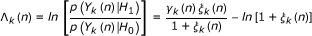(3)

where ξ k (n) is λS, k N, k representing the a priori signal-to-noise ratio (SNR) and γ k (n) is |Y k (n)|2 N, k denoting the a posteriori SNR. λ S, k and λ N, k should be estimated and the well-known method for estimating the a priori SNR is the decision-directed (DD) method  which is given as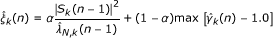(4)

where α is the weighting term, e.g., 0.98,is an estimate for the short-time power spectrum of clean speech derived from the minimum mean square error short-time spectral amplitude (MMSE-STSA) estimator ,, andis the estimated noise variance which is given by  as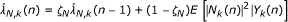(5)

where 0 < ζ N < 1 is the smoothing parameter, E[·] the expectation operator, and |N k (n)|2 the noise power spectrum. In Equation 5, the expectation term is also given by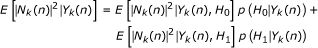(6)

Where(7)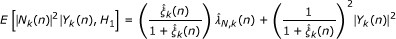(8)

In Equation 6, p(H0|Y k (n)) is the SAP at the k th frequency bin and derived from the Bayes' rule such that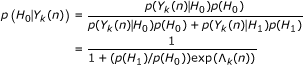(9)

with p(H0) representing the a priori probability of speech absence which is set to 0.2 in our case.

With the estimated parameters, the decision rule of SMVAD is given by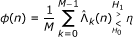(10)

whereis the LLR utilizingandand η is the decision threshold.

## 3. Analysis of LRT-based decision rule

In general, the LRT-based decision rule of SMVAD overlooks two undesirable problems. The first is that LRs cannot always show high values even if the input signal contains speech. Because of the basic assumption that noise is uncorrelated with speech, the complex Gaussian models of the input signal must satisfy the following condition:(11)

With condition (11), the peak of p(Y(n)|H1) is always lower than or equal to that of p(Y(n)|H0). Thus, p(Y(n)|H1) cannot always larger than p(Y(n)|H0) even in the case of speech presence. Therefore, an increased variance does not guarantee an increased LLR values. Figure 1 shows an example of this case with the three complex Gaussian PDFs having different variances. In Figure 1, the dotted line indicates the PDF only with noise variance, and the dashed and the solid lines represent the PDFs for which the a priori SNRs are -10 and 5 dB with the given noise variance, respectively.

Figure 2 shows two LLR curves related to Figure 1 with respect to the spectral amplitude of input signals where the dashed line indicates the LLR with the lower a priori SNR and the solid line represents the LLR with the higher a priori SNR, respectively. In Figure 2, the dashed circles show the difference between two LLRs for a low- and high-powered spectra at the given frequency bin. In the case of the left circle, there is a small difference between the solid and the dashed lines, and the solid line may be rather lower than the dashed line, even though the given a priori SNRs show a substantial difference. On the other hand, the right circle shows a large difference between the two LLR lines.

By inspecting the two cases, it is observed that the spectral power of input signals plays an important role in making the decision rule have a better discriminative property, because the conventional decision rule of the SMVAD was the average of all LLRs. In other words, the accuracy of the decision rule may be degraded by the LLRs derived from low-powered input spectrum.

Figure 3 shows an example of the undesirable cases of LLRs in a speech frame caused by both high a priori SNR and low-spectral power. In Figure 3a, x-axis represents the frequency bin index, the solid line indicates the spectral power of noisy speech, and the dotted line represents the estimated noise variance. As shown in Figure 3b, even though the a priori SNR is estimated to be high in this speech frame, it causes low LLR values when the input signal power is lower than the estimated noise variance. If the LLRs shown in Figure 3c are employed for decisions in SMVAD, this speech frame could not be detected as a speech frame. In Figure 3c, it is also observed that most of LLRs are close to 0 and high LLRs are located on the most high-powered frequency region. From the investigation of the first problem, it is concluded that the decision rule of SMVAD uses LLR at every frequency bin but not all of them contribute to a correct decision in case of the components in the low-powered frequency region.

The second problem of SMVAD also occurs on the low-powered frequency region of the noise. As mentioned in Section 1, the basic assumption on SMVAD is that noise is stationary over a long period of time, but, in practice, most of real noise powers tend to change slightly frame-by-frame. To accommodate this phenomenon, the estimated noise variance,, needs to be re-considered. Since the fixed smoothing parameter ζ N is generally chosen to be very close to 1, estimated noise variancechanges very smoothly. By this effect, estimated noise variancecan keep the a priori SNRvery low along the noise-only frames. As a result, the LLR with estimated SNRs can be simplified by(12)

Because of the smoothing operation, the a priori SNRcan be kept low or change very smoothly over the non-speech region. However, the a posteriori SNRand is greatly influenced by the unstable property of noise when noise is not stationary. Actually, the variations in the noise spectrum are not so serious in terms of the actual absolute value of noise and this phenomenon is good for estimating the reliable noise variance. However, since the a posteriori SNR is the ratio of the varying input spectrum |Y k (n)|2 to the almost fixed noise variance, the a posteriori SNRs in the low-powered frequency regions are more difficult to be settled on fixed low values. Figure 4 shows an example of the transition of a posteriori SNR with two different noise variances where y-axis means the ratio, (Noise variance + Varying Range)/Noise variance, which corresponds to the a posteriori SNR.

As shown in Figure 4, when the noise variance is very small, the transition of the a posteriori SNR is rapidly increased over the same varying range against the transition of the high noise variance. In the noise-only frames, the average of LLRs has to be close to 0. However, the a posteriori SNRs in low-powered frequency region can be high and possibly make certain LLR values as high as the levels in the speech frame. Using these LLRs in the decision rule, some of these noise frames can be detected as speech frames. Figure 5, where x-axis represents frequency bin, shows an actual case of the average car noise spectrum and the variance of a posteriori SNR according to the noise variance estimated on each frame. As already mentioned, in most high-powered frequency regions, the variances of a posteriori SNR are very close to 0, which means that there are low possibilities of high LLR values. In a low-powered region, on the contrary, higher variances may be shown. In case of (12), this effect brings about high a posteriori SNR which causes high LLRs.

In summary, if the input signal includes the speech signal, the LLRs in the low powered region could not be reliable because there is no way to judge whether the LLRs are caused by the speech signal or varying noise spectral components. Therefore, LLRs in the high-powered region are more important to let decision rule have an enhanced discriminative property. Figure 6 shows an actual case that SMVAD can save a speech frame when the decision rule of SMVAD only uses the LLRs in the high-powered region. In Figure 6, if we average all LLRs for decision, we could never detect the speech frame plotted in Figure 6a. On the contrary, based on our analysis, if we select or properly weight the LLRs for the decision rule, we can detect the speech frame because all of LLRs in low-powered region in Figure 6b can be excluded from the decision or reduced by the proper weights which can attenuate the effects of unreliable LLRs.

## 4. Modified decision rules

### 4.1. Selection of reliable LRs

By considering two undesirable phenomena analyzed in Section 3, it is discovered that LLRs in the high-powered frequency region are more reliable than those in the low-powered region. However, the concept for the high-powered region is still ambiguous for the time-varying input frames. In case of additive noise, since the average noise spectrum is almost fixed, the high-powered region may also be fixed for every noise frame, but in case of speech frames, the high-powered region can be moved by speech signals. Therefore, we need to find the high-powered region independently from the neighboring frames and only consider the total power of the current frame. Here, three assumptions for the selection of reliable LLRs are used:

1. 1.

The property of noise is mainly dependent on high-powered but less varying frequency components for which LLRs can be kept low.

2. 2.

Most of noise spectral power is concentrated on the high-powered region, irrespective of the existence of speech component.

3. 3.

When speech component exists in the current frame, the LLRs in the high-powered frequency region obtained because of the speech component may show high value.

Therefore, we propose two modified decision rules by selecting the frequency bins with reliable LLRs on the basis of the spectral power. At first, we reorder the input signal vector in terms of the spectral power such as Y(R)(n) = [Y(1)(n), Y(2)(n),...,Y(M)(n)] where |Y(r)(n)|2 ≥ |Y(s)(n)|2 for r > s and we also define LLR vector,where each elementis related to its corresponding Y(r)(n). With this vector, the first modified decision rule is defined as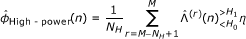(13)

where N H denotes the number of LR selected by the spectral power of frequency bins. By this decision rule, we can only consider the LLRs related to high-power frequency bins and N H is determined, empirically.

The second method is to compare the bin-power with the average power in each frame. Based on this idea, the second modified decision rule is given by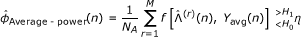(14)(15)

where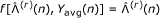if |Y(r)(n)|2Yavg(n), and f(r)(n), Yavg(n)] = 0 otherwise, and N A is the number of spectral components greater than or equal to the average power of each frame. In this method, we assumed that the spectral power in the high-powered region of noise is always greater than the frame average power.

### 4.2. Weighting scheme considering reliability of LRs

With the analysis of LRT-based decision rule, we also propose a weighting scheme to reflect the reliability of each LLR. As mentioned in Section 3, since the LLRs in the low-powered region of noise are not reliable because of the variation of the a posteriori SNR, it is desirable to consider more importantly the spectral powers of noisy speech which are much higher than the noise variance. In addition, as the noise variance becomes closer to the highest value of the noise variances at the current frame, the LLRs derived from the a posteriori SNRs would be reliable. Thus, the weights applied to each LLR are defined by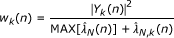(16)

whereis equal to the highest variance of the variance vectorwhich is composed ofs at all k th bins. In (16), each w k (n) can reduce the effects of the unstable a posteriori SNRs and cause LLRs in the high-powered region to remain on their own values or to increase. Thus, the new decision rule with this weight is given by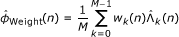(17)

## 5. Experiments

In the experiments, test data were composed of 60-s long speech data from the IEEE sentence listed in Table 1 and noise data from the AURORA database. The speech data were spoken by three male and three female speakers and sampled at 8 kHz. We used 20 ms frame size and 10 ms frame shift size. VAD decision was made every frame. The test material was all hand-labeled and consisted of 67% of speech and 33% of silence frames. For these experiments, we also used three types of noises, such as car, babble, and street noises at 5, 10, and 15 dB SNRs, respectively.

To compare with the proposed methods, we used four conventional methods as baseline systems. The first method is the typical SMVAD proposed by Sohn et al.  as described in Section 2, and the second method is SMVAD with the HMM hangover scheme which is specified in . The third conventional method is the DWT scheme proposed in . For training and testing of the DWT method, we used same parameters specified in . The experiment of DWT scheme was performed with six sets of 10-s long data used for testing and the remaining data used for training by the round-robin test. The fourth method is SMVAD using a multiple observation LRT (MO-LRT) proposed in . For the fourth method, we used one frame, which was experimentally chosen, before and after the current frame which is going to be determined as the speech or the non-speech.

The proposed methods are all evaluated by receiver operating characteristic (ROC) curves which show discriminative properties of VAD between noise-only and noisy speech frames in terms of the speech detection rate (SDR) and false-alarm rate (FAR) such that(18)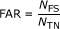(19)

where NCS, NTS, NFS, and NTN denote the number of correctly detected speech frames, total speech frames, falsely detected speech frames in silence regions, and total silence frames, respectively. In these experiments, we set N H = 10 forin (13).

In every ROC curve, HMM, DWT, MO, HP, AP, and weight in the parenthesis denote the results from the HMM hang-over scheme, the DWT scheme, the MO-LRT scheme, the first proposed decision rule in (13), the second proposed decision rule in (14), and the decision rule with the proposed weighting scheme, respectively. For practical comparison of performances, we focus on SDRs of the methods when FAR is low. We consider that the VAD can show a reasonable discriminating property when FAR < 0.2. In the ROC curves, the red lines represent the results of the proposed methods and the blue lines indicate the results of the conventional methods.

In the car noise environment of Figure 7, all of the proposed methods show better performance than the conventional methods do. In addition, the SDRs of SMVAD(HP) and SMVAD(Weight) are at least 0.1 higher than those of SMVAD when FAR < 0.05. Especially, SMVAD(Weight) keeps the highest SDR at the extremely low FAR.

In babble noise environment of Figure 8, every proposed method also shows better performance than the conventional methods do as in the car noise environment, but the difference is that the performance improvement of SMVAD(HP) and (AP) are not noticeable. However, we can also observe that the performance improvement of SMVAD(Weight) is kept constant as the SNR becomes higher and can be still considered to be significant.

In street noise environment of Figure 9, SMVAD(Weight) is effective on improving the performance of SMVAD and shows significantly higher SDR at FAR = 0.05 with 5 dB SNR than SMVAD(HMM). In case of 10 dB SNR, the performance of SMVAD(HMM) is almost the same as SMVAD(HP) or SMVAD(AP), but it is still not better than that of SMVAD(Weight).

From the investigation of the experimental results, it is observed that SMVAD(Weight) shows the highest and the most consistent performance improvement in all noise conditions. In addition, SMVAD(DWT) did not show better performance than SMVAD(Weight) does although the complexity of SMVAD(Weight) is almost the same as that of SMVAD. By these results, we can conclude that the variation of input signal statistics has a large influence on the accuracy of VAD, and if we are not sure to know about specific noise type, it would be better to use SMVAD(Weight) for stable performance improvement under unexpected noise environments.

## 6. Conclusion

In this article, we introduced SMVAD, and analyzed the averaged LRT-based decision rule and its undesirable phenomena which can possibly happen in various noise environments. To reduce the undesirable phenomena, we proposed two types of modified decision rules based on the selection of reliable LRs and a weighting scheme applied to LLRs used in the decision rule. Compared with the conventional methods, it was proved that the proposed methods are much more robust in various noise environments without any training procedure and additional computational complexity. Among the proposed methods, SMVAD(Weight) showed the most reliable performance improvement under various conditions.

For further studies, the properties of speech and noise, which can be applied to the weights for LLRs, are needed to be analyzed in more details.

## Abbreviations

DWT:

discriminative weight training

FAR:

false-alarm rate

HMM:

hidden Markov model

LRs:

likelihood ratios

LRT:

likelihood ratio test

MMSE-STSA:

minimum mean square error short-time spectral amplitude

MO-LRT:

multiple observation LRT

PDF:

probability density function

ROC:

SAP:

speech absence probability

SDR:

speech detection rate

SLRs:

smoothed likelihood ratios

statistical model-based voice activity detector

SNR:

signal-to-noise ratio

voice activity detector.

## References

1. 1.

Srinivasan K, Gersho A: Voice activity detection for cellular networks. Proc IEEE Speech Coding Workshop 1993, 85-86.

2. 2.

Sohn J, Sung W: A voice activity detector employing soft decision based noise spectrum adaptation. Proc Int Conf Acoustics, Speech, and Signal Processing 1998, 365-368.

3. 3.

Sohn J, Kim NS, Sung W: A statistical model-based voice activity detection. IEEE Signal Process Lett 1999,6(1):1-3. 10.1109/97.736233

4. 4.

Cho YD, Kondoz A: Analysis and improvement of a statistical model-based voice activity detector. IEEE Signal Process Lett 2001,8(10):276-278. 10.1109/97.957270

5. 5.

Chang J-H, Kim NS, Mitra SK: Voice activity detection based on multiple statistical models. IEEE Trans Signal Process 2006,54(6):1965-1976.

6. 6.

Kang S-I, Jo Q-H, Chang J-H: Discriminative weight training for a statistical model-based voice activity detection. IEEE Signal Process Lett 2008, 15: 170-173.

7. 7.

Ramírez J, Segura JC, Benítez C, García L, Rubio A: Statistical voice activity detection using a multiple observation likelihood ratio test. IEEE Signal Process Lett 2005,12(10):689-692.

8. 8.

Kim Y-G, Suh Y-J, Kim H-R: Selection of reliable likelihood ratios for statistical model-based voice activity detection. Proc of Asia Pacific Signal and Information Processing Association Annual Summit and Conf. 2009, CD-ROM 2009.

9. 9.

Ramírez J, Segura JC, Benítez C, Torre Á, Rubio AJ: A new Kullback-Leibler VAD for speech recognition in noise. IEEE Signal Process Lett 2004,11(2):266-269. 10.1109/LSP.2003.821762

10. 10.

Garner PN, Fukada T, Komori Y: A differential spectral voice activity detector. Proc Int Conf Acoustics, Speech, and Signal Processing 2004, 597-600.

11. 11.

Davis A, Nordholm S, Togneri R: Statistical voice activity detection using low-variance spectrum estimation and an adaptive threshold. IEEE Trans Audio Speech Lang Process 2006,14(2):412-424.

12. 12.

Shin JW, Kwon HJ, Jin SH, Kim NS: Voice activity detection based on conditional MAP criterion. IEEE Signal Process Lett 2008, 15: 257-260.

13. 13.

Ramírez J, Górriz JM, Segura JC, Puntonet CG, Rubio AJ: Speech/non-speech discrimination based on contextual information integrated bispectrum LRT. IEEE Signal Process Lett 2006,13(8):497-500.

14. 14.

Chang J-H, Shin JW, Kim NS: Voice activity detector employing generalised Gaussian distribution. Electron Lett 2004,40(24):1561-1563. 10.1049/el:20047090

15. 15.

Ephraim Y, Malah D: Speech enhancement using a minimum mean-square error short-time spectral amplitude estimator. IEEE Trans Acoust Speech Signal Process 1984,ASSP-32(6):1109-1121.

16. 16.

Kim NS, Chang J-H: Spectral enhancement based on global soft decision. IEEE Signal Process Lett 2000,7(5):108-110. 10.1109/97.841154

## Acknowledgements

This study was supported by the NRF grant funded by the Korean government (MEST) (No. 2011-0017967).

## Author information

Authors

### Corresponding author

Correspondence to Youngjoo Suh.

### Competing interests

The authors declare that they have no competing interests.

## Authors’ original submitted files for images

Below are the links to the authors’ original submitted files for images.

## Rights and permissions

Reprints and Permissions

Kim, Y., Suh, Y. & Kim, H. Reliable likelihood ratios for statistical model-based voice activity detector with low false-alarm rate. EURASIP J. Adv. Signal Process. 2011, 31 (2011). https://doi.org/10.1186/1687-6180-2011-31